Скачать презентацию Image segmentation by clustering in the color space

eaa14f71b423188ac167863867006290.ppt

• Количество слайдов: 22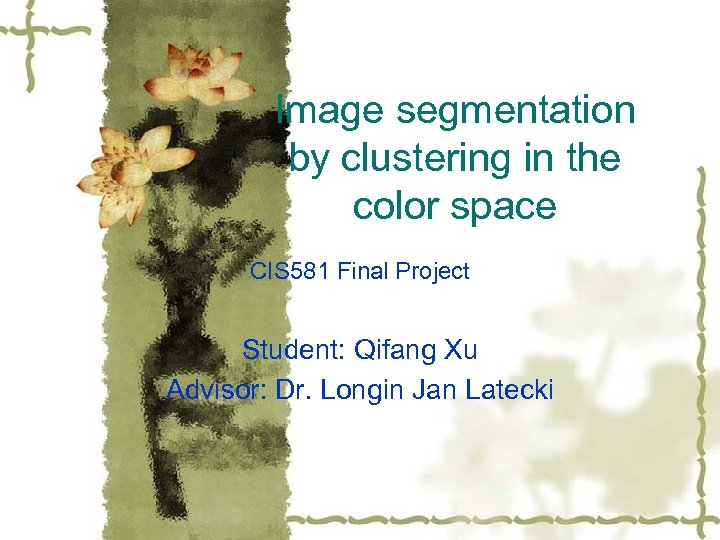Image segmentation by clustering in the color space CIS 581 Final Project Student: Qifang Xu Advisor: Dr. Longin Jan Latecki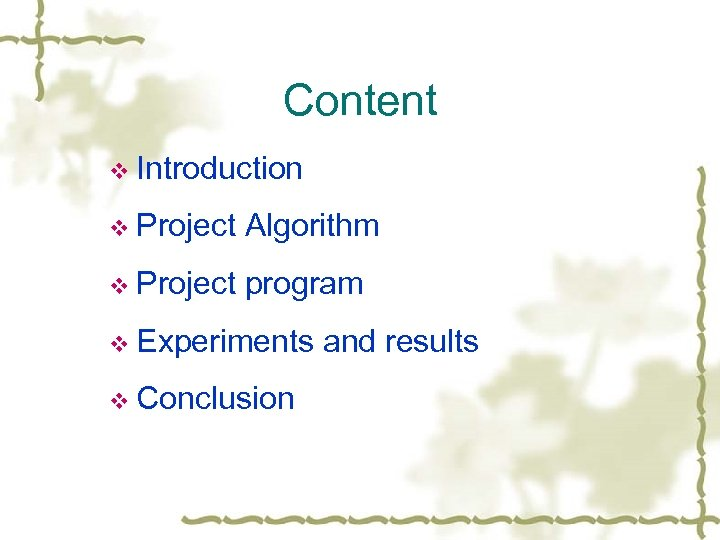Content v Introduction v Project Algorithm v Project program v Experiments v Conclusion and results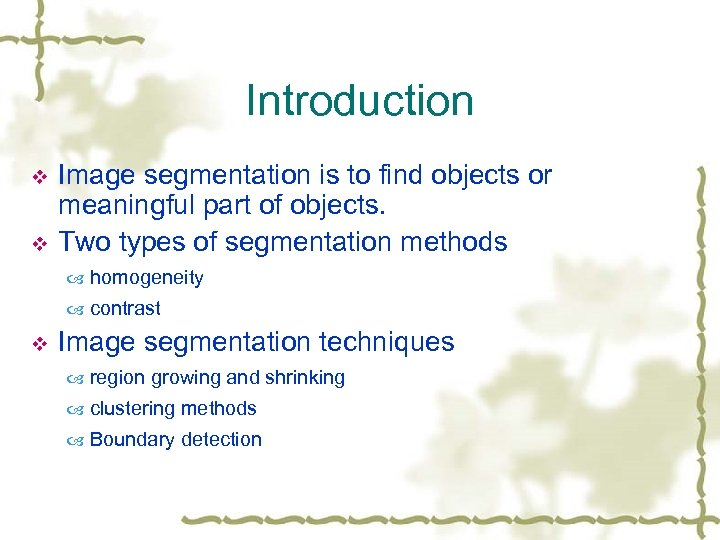Introduction v v Image segmentation is to find objects or meaningful part of objects. Two types of segmentation methods homogeneity contrast v Image segmentation techniques region growing and shrinking clustering methods Boundary detection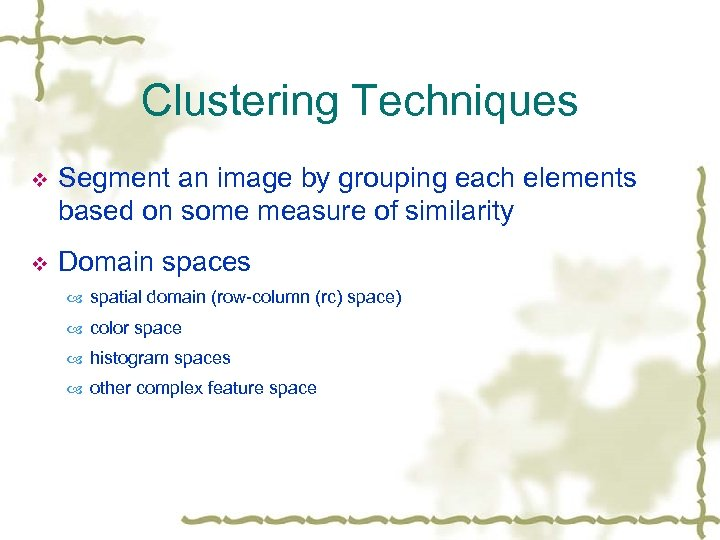Clustering Techniques v Segment an image by grouping each elements based on some measure of similarity v Domain spaces spatial domain (row-column (rc) space) color space histogram spaces other complex feature space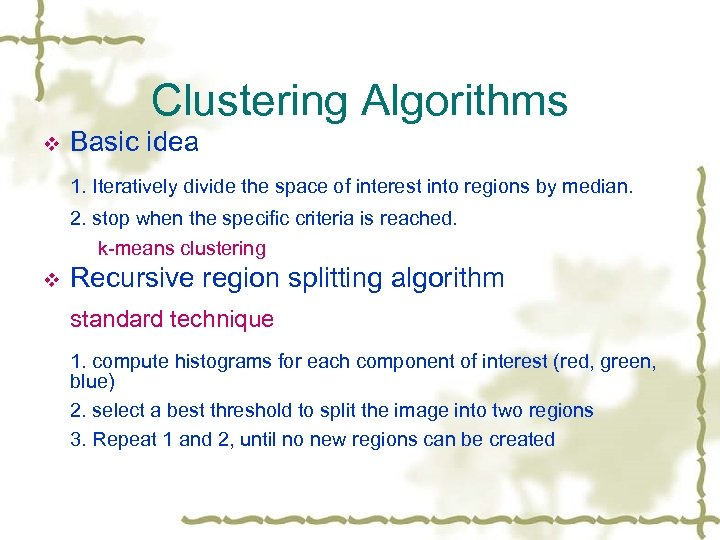Clustering Algorithms v Basic idea 1. Iteratively divide the space of interest into regions by median. 2. stop when the specific criteria is reached. k-means clustering v Recursive region splitting algorithm standard technique 1. compute histograms for each component of interest (red, green, blue) 2. select a best threshold to split the image into two regions 3. Repeat 1 and 2, until no new regions can be created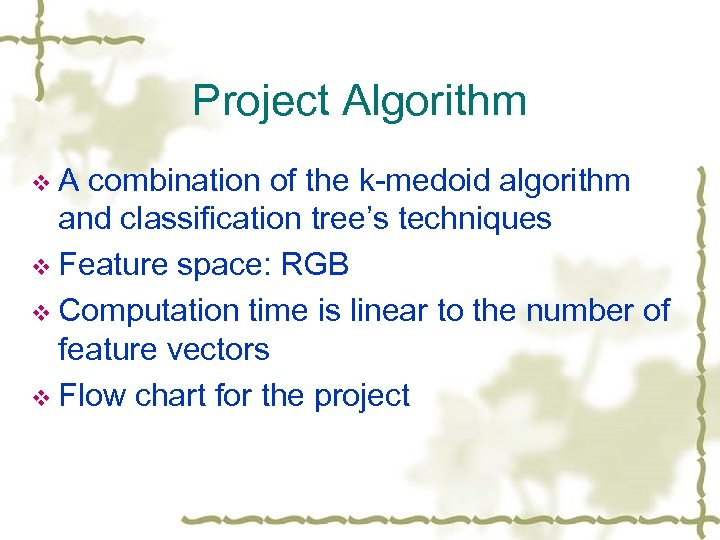Project Algorithm v. A combination of the k-medoid algorithm and classification tree’s techniques v Feature space: RGB v Computation time is linear to the number of feature vectors v Flow chart for the project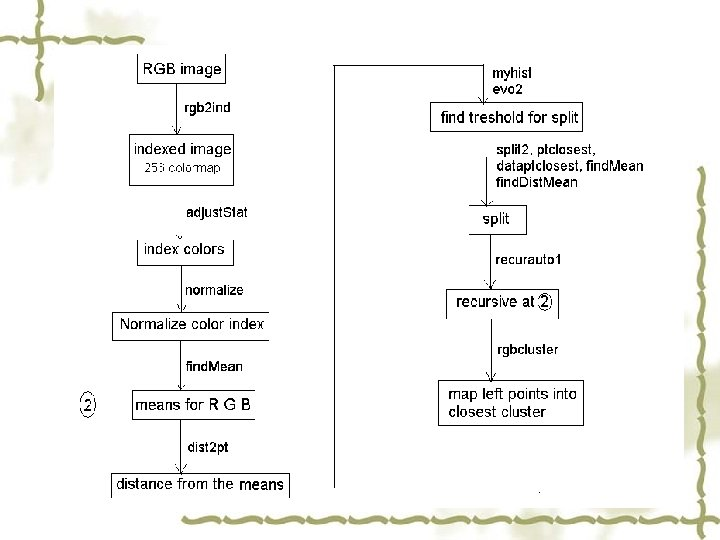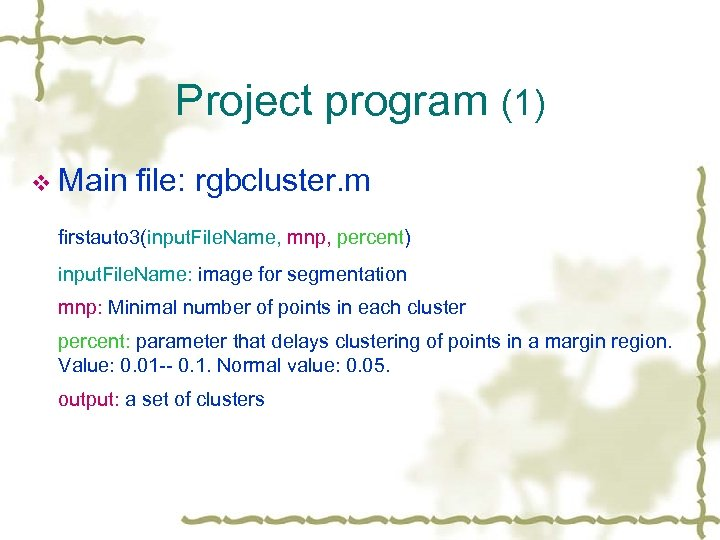Project program (1) v Main file: rgbcluster. m firstauto 3(input. File. Name, mnp, percent) input. File. Name: image for segmentation mnp: Minimal number of points in each cluster percent: parameter that delays clustering of points in a margin region. Value: 0. 01 -- 0. 1. Normal value: 0. 05. output: a set of clusters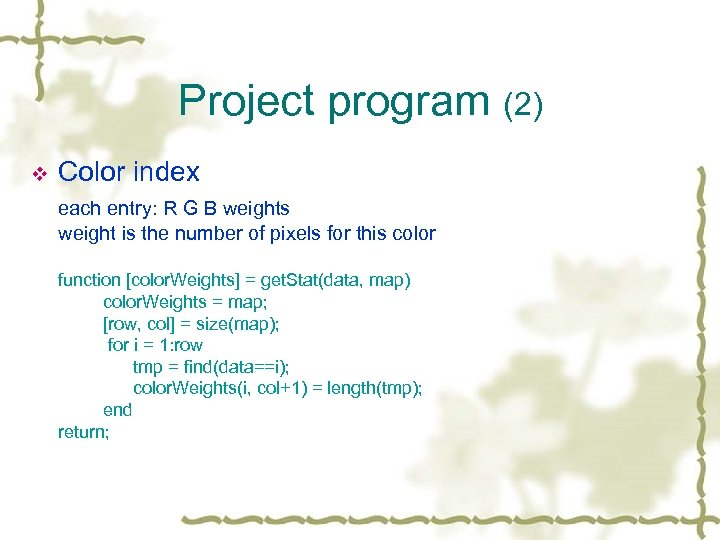Project program (2) v Color index each entry: R G B weights weight is the number of pixels for this color function [color. Weights] = get. Stat(data, map) color. Weights = map; [row, col] = size(map); for i = 1: row tmp = find(data==i); color. Weights(i, col+1) = length(tmp); end return;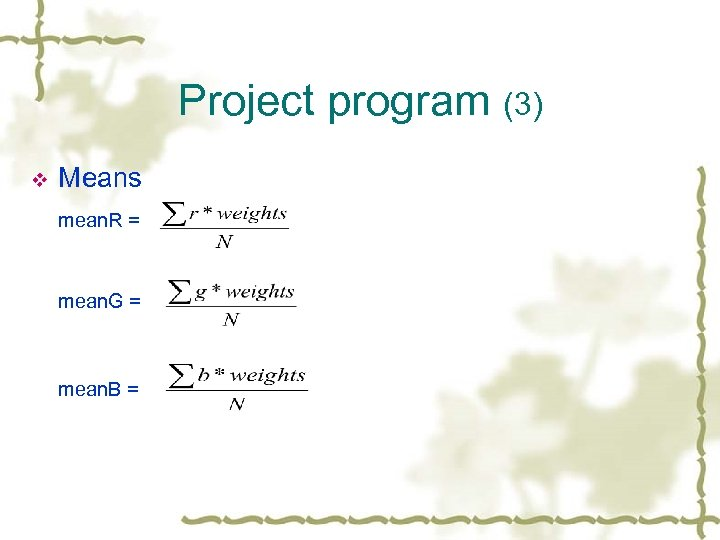Project program (3) v Means mean. R = mean. G = mean. B =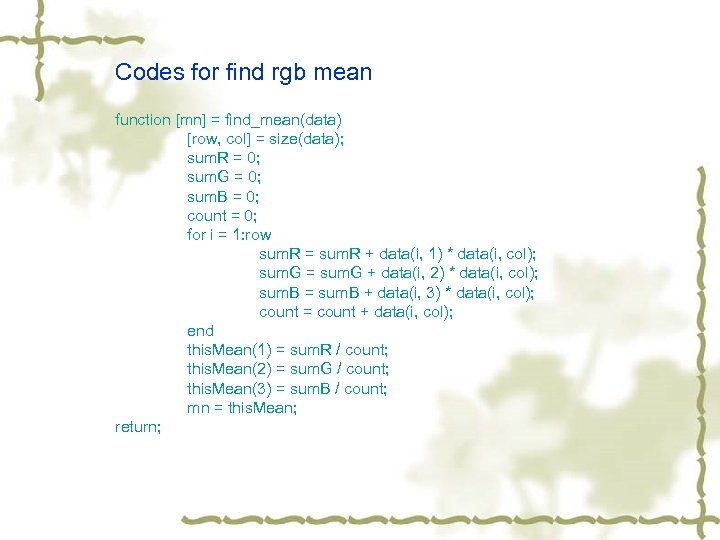Codes for find rgb mean function [mn] = find_mean(data) [row, col] = size(data); sum. R = 0; sum. G = 0; sum. B = 0; count = 0; for i = 1: row sum. R = sum. R + data(i, 1) * data(i, col); sum. G = sum. G + data(i, 2) * data(i, col); sum. B = sum. B + data(i, 3) * data(i, col); count = count + data(i, col); end this. Mean(1) = sum. R / count; this. Mean(2) = sum. G / count; this. Mean(3) = sum. B / count; mn = this. Mean; return;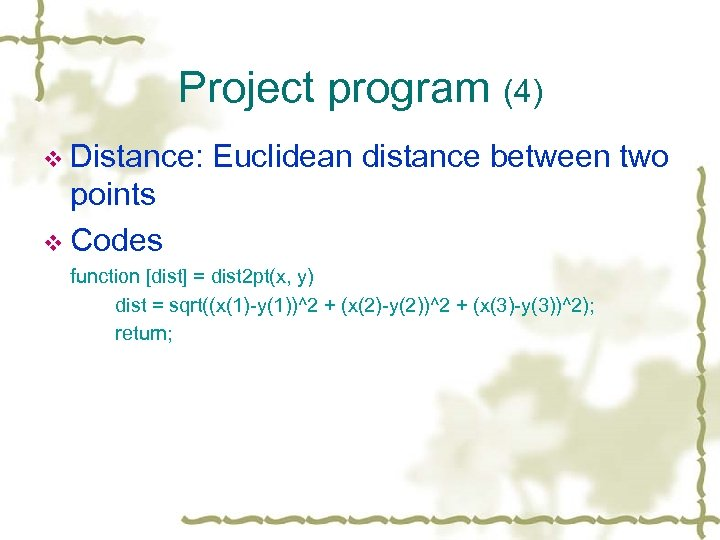Project program (4) v Distance: Euclidean distance between two points v Codes function [dist] = dist 2 pt(x, y) dist = sqrt((x(1)-y(1))^2 + (x(2)-y(2))^2 + (x(3)-y(3))^2); return;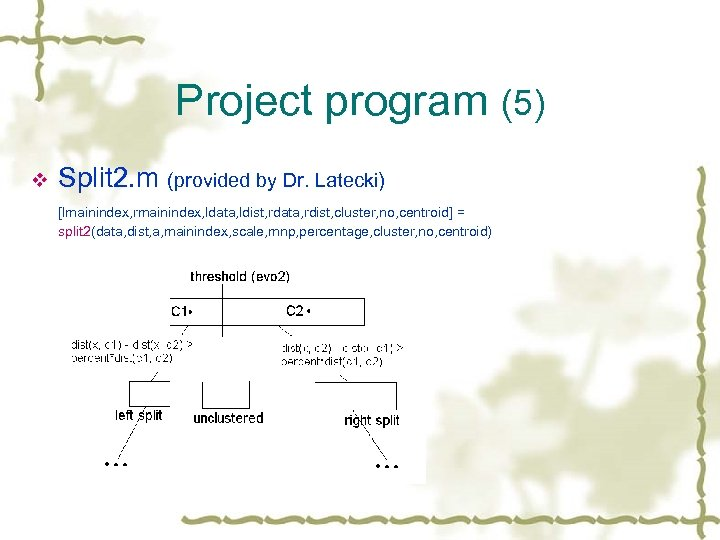Project program (5) v Split 2. m (provided by Dr. Latecki) [lmainindex, rmainindex, ldata, ldist, rdata, rdist, cluster, no, centroid] = split 2(data, dist, a, mainindex, scale, mnp, percentage, cluster, no, centroid)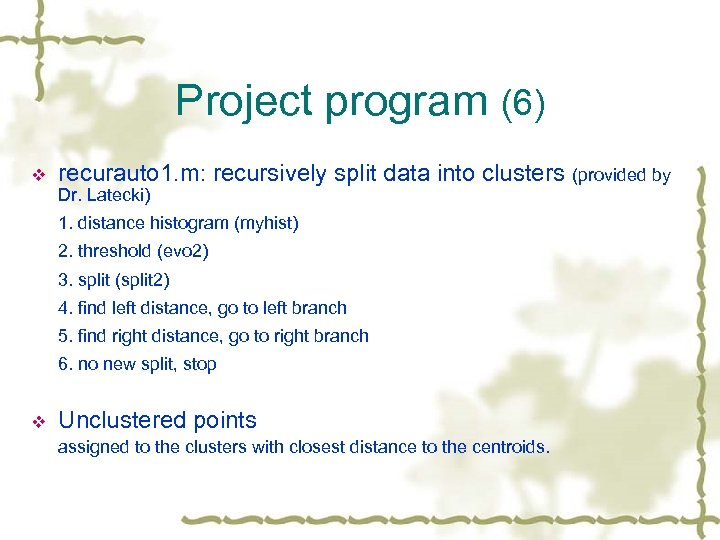Project program (6) v recurauto 1. m: recursively split data into clusters (provided by Dr. Latecki) 1. distance histogram (myhist) 2. threshold (evo 2) 3. split (split 2) 4. find left distance, go to left branch 5. find right distance, go to right branch 6. no new split, stop v Unclustered points assigned to the clusters with closest distance to the centroids.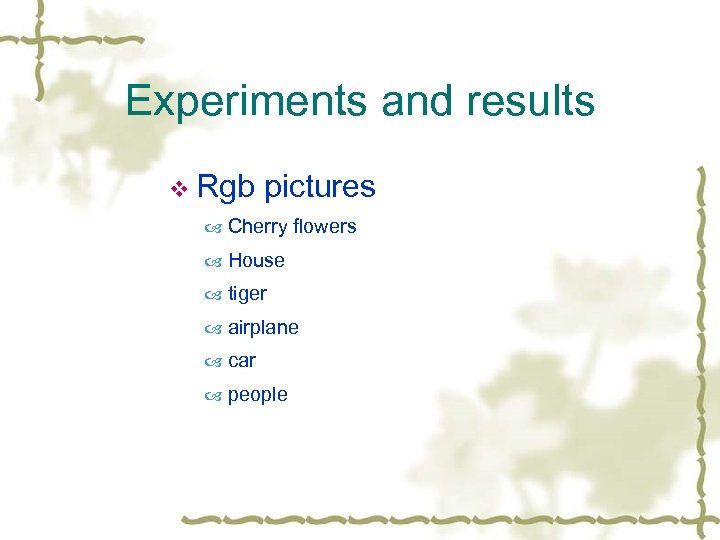Experiments and results v Rgb pictures Cherry flowers House tiger airplane car people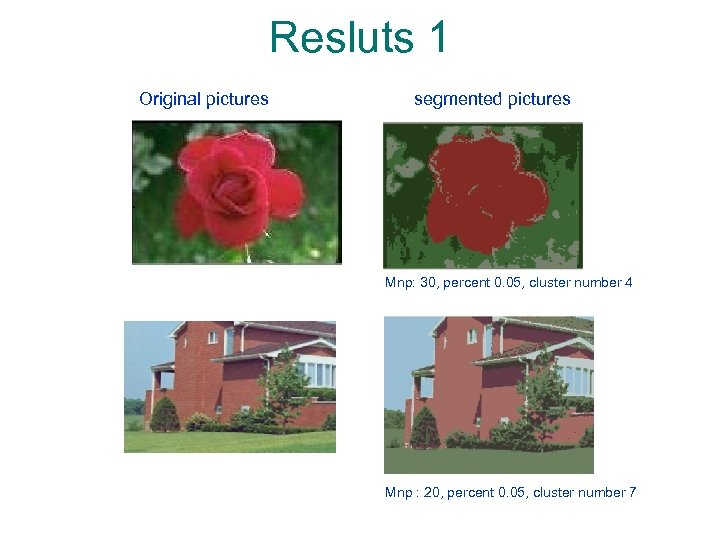Resluts 1 Original pictures segmented pictures Mnp: 30, percent 0. 05, cluster number 4 Mnp : 20, percent 0. 05, cluster number 7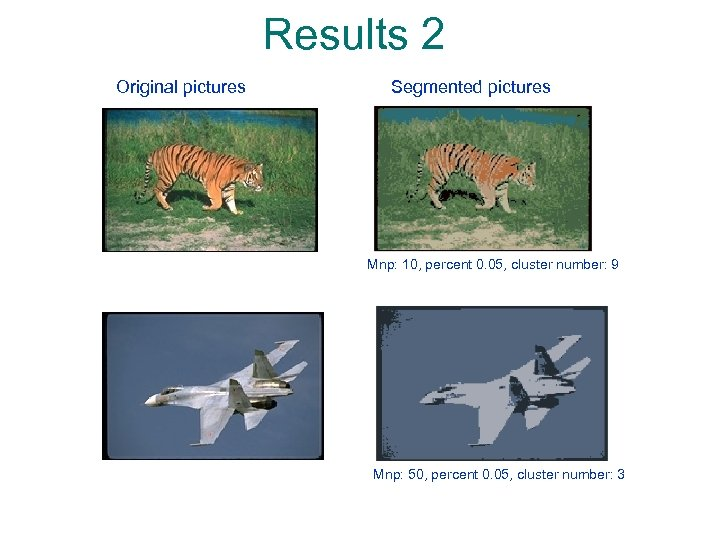Results 2 Original pictures Segmented pictures Mnp: 10, percent 0. 05, cluster number: 9 Mnp: 50, percent 0. 05, cluster number: 3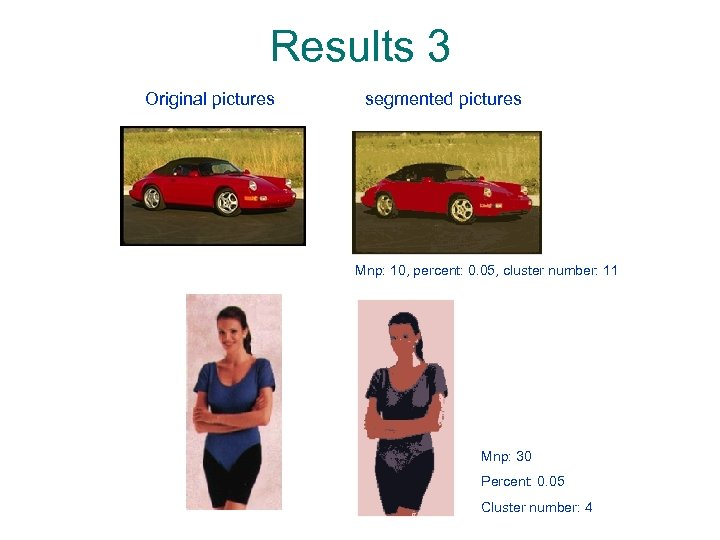Results 3 Original pictures segmented pictures Mnp: 10, percent: 0. 05, cluster number: 11 Mnp: 30 Percent: 0. 05 Cluster number: 4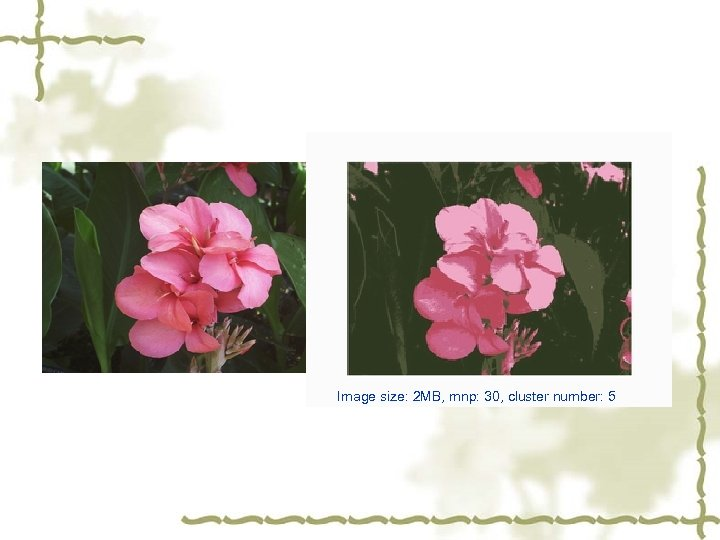Image size: 2 MB, mnp: 30, cluster number: 5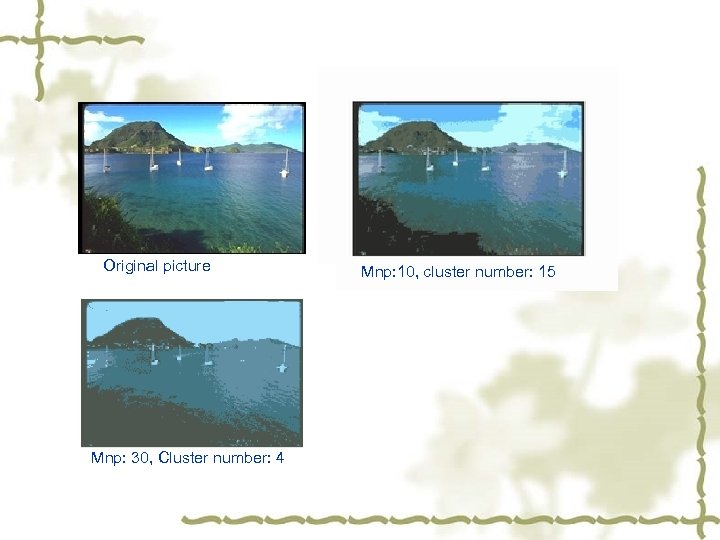Original picture Mnp: 30, Cluster number: 4 Mnp: 10, cluster number: 15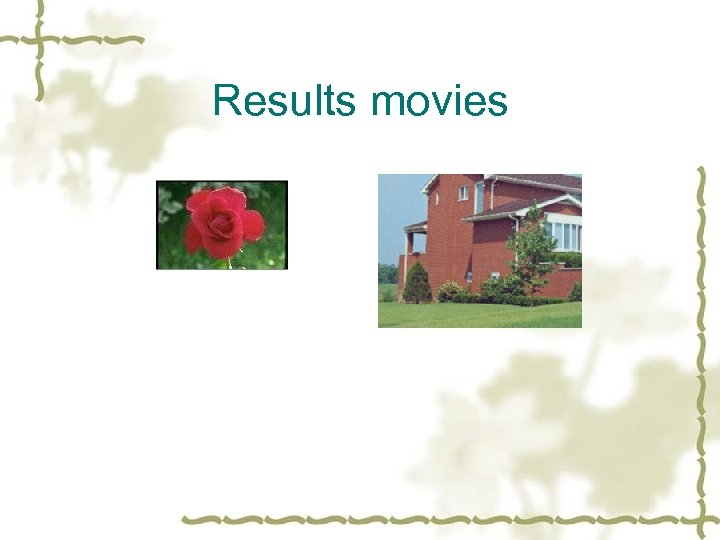Results movies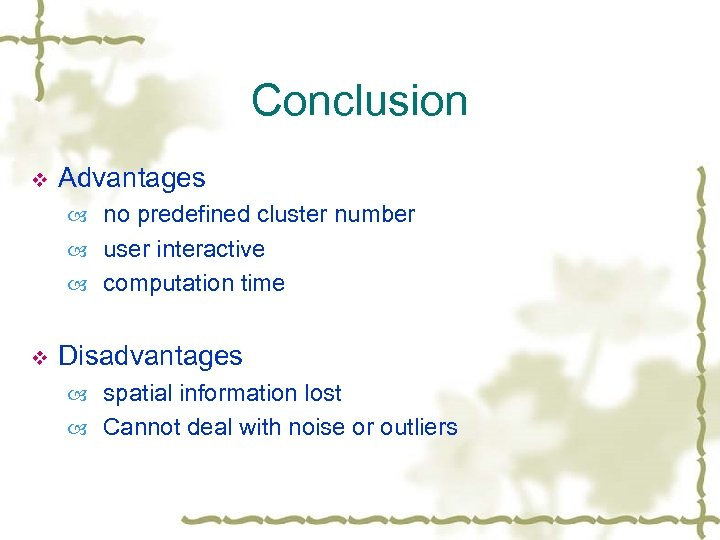Conclusion v Advantages no predefined cluster number user interactive computation time v Disadvantages spatial information lost Cannot deal with noise or outliers